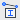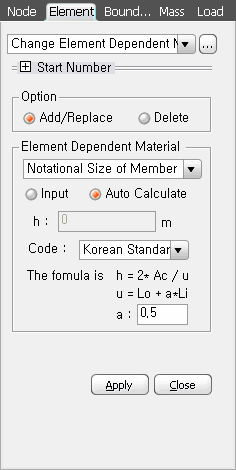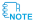Material Properties Created Edited

# Change Property

## Function

• To consider the effect of creep/shrinkage on concrete elements over time, you need to input the geometric dimensions. The geometric dimensions may vary depending on the extent of exposure of the cross-section to the environment. If you have multiple cross-sections, you should input the corresponding geometric dimensions for each element.
• By using this feature, if you input geometric dimensions for each element, the geometric dimensions specified in the Time Dependent Material (Creep/Shrinkage) input will be disregarded.
• This feature is not applicable in the Construction Stage Composite Section, and you need to directly input the geometric dimensions for each part in the Composite Section for Construction Stage.

## Call

From main menu, select [Properties] tab > [Time Dependent Material] group >[Change Property]

## Input### Option

Delete : Delete the geometric shape dimension (h).

### Element Dependent Material

Notational Size of Member

Input : Specify the user-defined h

Auto Calculate : The Program auto-calculates the values using the section properties

Code : Select the applicable code or standard for calculating the geometric dimensions (h)

a : Enter "a"span style="font-weight: bold;">a"according to a standard.

u = Lo + a*Li

Lo : The perimeter length of a box-shaped section in contact with the external atmosphere

Li : The perimeter length of a box-shaped section in contact with the internal atmosphere1. When using this function to change the geometric dimensions, the geometric dimensions (h) inputted in "Time Dependent Material (Creep/Shrinkage)" will be disregarded, and the values inputted in the change will take precedence.

2. If using the Composite Section for Construction Stage, the "h" values for each part should be directly inputted in the Composite Section for Construction Stage.

Volume Surface Ratio

Input : You can directly input the geometric dimensions to be applied to the selected element.

Auto Calculate : You can calculate the geometric dimension (h) using the provided equation from the selected standard.

Code : Select the standard to be applied when calculating the geometric dimension. (The formula is v/s = Volume/Structure in ACI, PCA, Combined(ACI&PCA) Code)

v : Volume

s : Surface

0